International Journal of Information and Communication Sciences
Volume 1, Issue 2, October 2016, Pages: 30-34

Spectrally Efficient SAC-OCDMA System Using a New Two-Code Keying Scheme

Hamza M. R. Al-Khafaji1, Razali Ngah2, Alaa J. Mousa3, S. A. Aljunid4

1Computer Techniques Engineering Department, Al-Mustaqbal University College, Babylon, Iraq

2Wireless Communication Centre, Faculty of Electrical Engineering, Universiti Teknologi Malaysia, UTM Skudai, Johor, Malaysia

3Computer Techniques Engineering Department, Islamic University College, Najaf, Iraq

4School of Computer and Communication Engineering, Universiti Malaysia Perlis, Perlis, Malaysia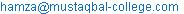(H. M. R. Al-Khafaji)

Hamza M. R. Al-Khafaji, Razali Ngah, Alaa J. Mousa, S. A. Aljunid. Spectrally Efficient SAC-OCDMA System Using a New Two-Code Keying Scheme. International Journal of Information and Communication Sciences. Vol. 1, No. 2, 2016, pp. 30-34. doi: 10.11648/j.ijics.20160102.13

Received: July 2, 2016; Accepted: August 19, 2016; Published: September 26, 2016

Abstract: This paper introduces a new two-code keying scheme for increasing the spectral efficiency (SE) of spectral-amplitude-coding optical code-division multiple-access (SAC-OCDMA) system. The analytical expression is derived for the SE of SAC-OCDMA system based on the proposed scheme utilizing multi-diagonal (MD) code. The theoretical results demonstrate that the suggested scheme has an improved SE performance over the conventional unipolar encoding with direct detection technique. This SE enhancement is verified in simulation experiments.

Keywords: SAC-OCDMA, Spectral Efficiency, Two-Code Keying Scheme, Bipolar Encoding, MD Code

Contents

1. Introduction

Optical code-division multiple-access (OCDMA) has been considered as a viable option for implementing the next-generation optical access networks because of its attractive properties, such as high-level security during transmission, flexibility in code design, and possibility of plug and play functionality [1,2]. Unfortunately, OCDMA systems pose the crucial disadvantage of multiple-access interference (MAI), which restricts the number of concurrent subscribers and creates an asymptotic floor to the bit-error rate (BER) [3,4]. Recently, there has been increasing at dramatic rates of concern in spectral-amplitude-coding optical code-division multiple-access (SAC-OCDMA) systems due to mitigating abilities of MAI and cost savings [5,6]. Research has shown that MAI can be obviated in SAC-OCDMA systems by two ways: with fixed in-phase cross-correlation codes and subtraction detection techniques, or with zero cross-correlation (ZCC) codes and direct detection technique [5,7]. Still, they are suffered from phase-induced intensity noise (PIIN), which arises from the non-coherency of the broadband sources of various transmitters . PIIN is a limiting factor of the data rate, communication quality, and capacity of non-coherent SAC-OCDMA systems . There are various codes and detection techniques that have been proposed in the literature to solve the PIIN limitation in SAC-OCDMA systems. Of these, using a multi-diagonal (MD) code, which has ZCC and free cardinality attributes constitute a favourable method to diminish PIIN’s influence in SAC-OCDMA systems . In this paper, a new two-code keying approach is proposed for spectral efficiency (SE) enhancement in SAC-OCDMA systems based on MD code. Succeeding the introduction in Section 1, Section 2 designates the suggested scheme. Subsequently, the SE analysis of SAC-OCDMA system based on the proposed scheme with MD code, supported with theoretical and simulation results are presented in Section 3. Lastly, the conclusion is given in Section 4.

2. Description of the Suggested Scheme

Figure 1 illustrates the structure of the SAC-OCDMA transmitter and receiver based on the proposed two-code keying scheme. The SAC-OCDMA network considered here consists of several transmitters and receivers positioned in the periphery of a star network configuration. The modulated optical signal from the light-emitting diode (LED) is filtered by the encoders with different MD codes according to the data bit of every user. The encoders split the optical bandwidth into bins and allocate MD codes to every user based on which set of bins is occupied. When bit ‘1’ is sent, light is transmitted to encoder 1, while bit ‘0’ is denoted by light transmitted to encoder 2. Encoded signals are delivered over the OCDMA network and assumed to arrive with equal power at the receivers. The received optical signal is spread to the decoders that have the similar spectral response as the encoders. Following the user signal is detected by the photodetector (PD), the transmitted information are restored and filtered by a low-pass filter (LPF).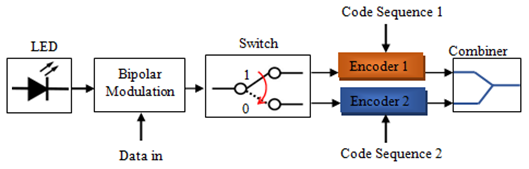(a)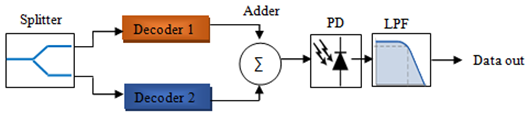(b)

(a) SAC-OCDMA transmitter; (b) SAC-OCDMA receiver.

Fig. 1. The structure of the suggested two-code keying scheme [11-14].

3. SE Analysis and Results

The power spectral densities (PSDs) when the wanted user is active are:(1)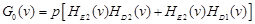(2)

where G1 (v) and G0 (v) represent the PSD at the PD when the wanted user is sending ‘1’ and ‘0’ bits, respectively. p is the received PSD level at the PD. HE1 (v), HE2 (v), HD1 (v), and HD2 (v) are encoder 1, encoder 2, decoder 1, and decoder 2 transfer functions, respectively.

The mean optical power reaching PD is computed by :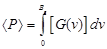(3)

Substituting (1) and (2) in (3), the mean optical power formulas are: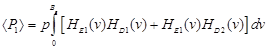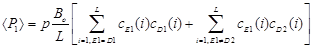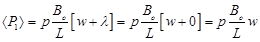(4)

Similarly,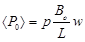(5)

where cE (i) and cD (i) represent the ith element of the encoders and decoders code words, respectively, Bo is the optical bandwidth which is equal to 3.75 THz, and l is the in-phase cross-correlation for MD code.

The relation among the code length L, the number of active users K, and the code weight w for MD code is expressed by :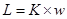(6)

The mean optical power of the wanted user is expressed by: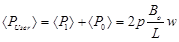(7)

The PIIN expressions can be expressed as follows :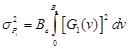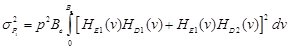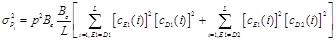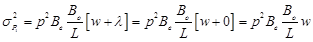(8)

Similarly,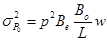(9)

where Be is the noise-equivalent electrical bandwidth.

The total PIIN of the wanted user is expressed by: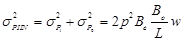(10)

The variance of shot noise is given by: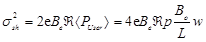(11)

where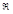is the PD’s responsivity which is equal to 0.75, and e is the electron’s charge 1.602 × 10-19 C.

The variance of thermal noise can be evaluated as: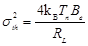(12)

where kB is the Boltzmann’s constant 1.38066 × 10-23 J/K, Tn is the receiver noise temperature equals to 300 K, and RL is the receiver load resistance which is equal to 1030 Ω.

The signal-to-noise ratio (SNR) is thus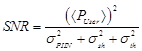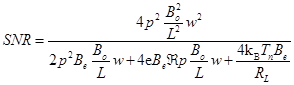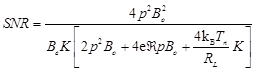(13)

Therefore, from (13), Be is thus: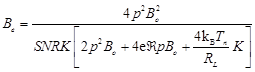(14)

Based on the approximation of Gaussian distribution, the BER is :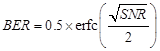(15)

where erfc is the complementary error function.

The SE (ηS) for a given BER is expressed as: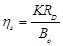(16)

Assuming Be = 0.75 × RD , where RD is the data rate, and substituting Be from (14) into (16), the derived SE formula is: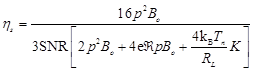(17)

Fig. 2 presents the SE with regard to the number of active users K for MD code at different BERs and received optical power (ROP) of −25 dBm. The proposed two-code keying scheme leads to a better SE performance than the existing unipolar encoding with direct detection technique. For BER=10–9, the suggested two-code keying scheme curve crosses K= 40 with 0.01658 b/s/Hz, whereas the conventional unipolar encoding with direct detection technique curve crosses K= 40 with 0.005385 b/s/Hz. This occurrence shows the proposed scheme provides 4.88 dB SE enhancement compared to the existing unipolar encoding with direct detection technique. Further, Fig. 2 shows that when the BER requirement of the system is decreased to 10–11, the SE is decreased. For BER=10–11, the recommended two-code keying scheme curve crosses K=40 with 0.01327 b/s/Hz, which shows a 0.96 dB SE advantage in case of BER = 10−9 against BER = 10−11. Moreover, it can be recognized that SE decreases with increasing number of active users. For BER=10–11, the unipolar encoding with direct detection technique curve crosses K=10 with 0.009085 b/s/Hz and K= 100 with 0.0021 b/s/Hz. This occurrence shows a 6.36 dB SE penalty for an increase of 90 users.

The SE is investigated through simulation experiments for 10 channels of MD code (w=4) utilizing OptiSystem software (version 13) from optiwaveTM. Separate LEDs with 3.75 THz optical bandwidth and 0 dBm launch power are used. The information signals are generated by using the pseudo random bit sequence generator with the non-return-to-zero line coding. A 30 km single-mode fiber is used with attenuation, resultant dispersion, and polarization-mode dispersion coefficients of 0.25 dB/km, 18 ps/nm∙km, and 0.2 ps/Ökm, respectively, at a wavelength of 1550 nm. The four-wave mixing and self-phase modulation are specified according to typical industry values. The noises generated at the receivers are random and totally uncorrelated. The dark current value is set at 5 nA, and the thermal noise coefficient is 1.8 × 10–23 W/Hz for each positive-intrinsic-negative PD. The cut-off frequency of the electrical LPFs is set at 0.75 of the data rate. The Gaussian algorithm is used for BER estimation. Fig. 3 compares the eye diagrams of MD code to elucidate the resultant SE. The eye diagrams show that the SE is enhanced using the proposed scheme owing to the increase in data rate without compromising the BER.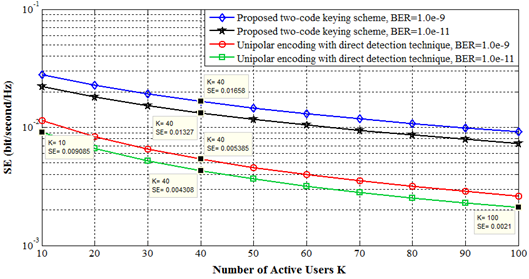Fig. 2. SE versus number of active users K at different BERs.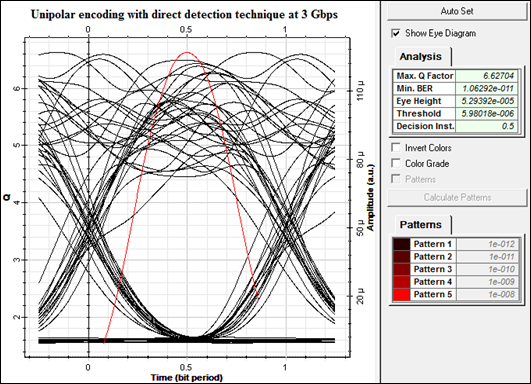(a)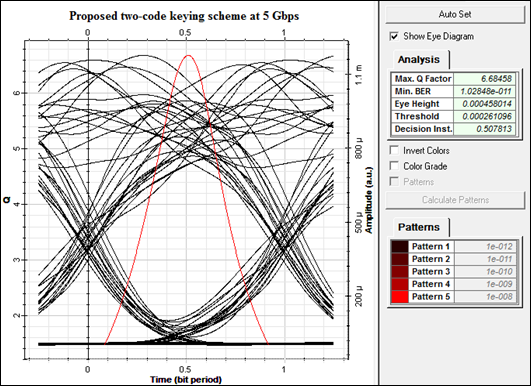(b)

Fig. 3. Eye diagrams for MD code using: (a) the suggested scheme at 5 Gbps; (b) the unipolar encoding with direct detection technique at 3 Gbps.

4. Conclusion

It is shown that the newly proposed two-code keying scheme enables bipolar encoding and increases the SE compared to the prior reported unipolar encoding with direct detection technique. Further, this design is appropriate with any codes that have ZCC attribute. The SAC-OCDMA system based on the recommended scheme is seen as a competitive solution for implementing future optical fiber networks.

References

1. Kitayama, K.I.:Optical code division multiple access: a practical perspective’,Cambridge University Press, 2014.
2. Kwong, W.C., and Yang, G.-C.:Optical coding theory with prime’,CRC Press Taylor & Francis Group, 1st ed., 2013.
3. Sahuguede, S., Julien-Vergonjanne, A., and Cances, J.P.: ‘Beat noise compensation in OCDMA systems using soft decoding based FEC’, IEEE Transactions on Communications, 2011, 59, pp. 1400−1410.
4. Hussein, G.A., Abd El-atty, S.M., Oraby, O.A., Mohamed, A.E.A., Elkorany, A.S., Eldokany, I., Dessouky, M.I., El-Rabaie, E.M., Alshebeili, S.A., and El-Samie, F.E.: ‘An efficient MAI cancellation technique in optical CDMA’,Optik-International Journal for Light and Electron Optics, 2014, 125, pp. 29953000.
5. Al-Khafaji, H.M.R., Aljunid, S.A., Amphawan, A., and Fadhil, H.A.: ‘Improving spectral efficiency of SAC-OCDMA systems by SPD scheme’, IEICE Electron. Express, 2012, 9, pp. 1829−1834.
6. Shastri, B.J., El-Sahn, Z.A., Zeng, M., Kheder, N., Rusch, L.A., and Plant, D.V.: ‘A standalone burst-mode receiver with clock and data recovery, clock phase alignment, and RS (255, 239) codes for SAC-OCDMA applications’,IEEE Photonics Technology Letters, 2008, 20, pp. 363365.
7. Anuar, M.S., Aljunid, S.A., Saad, N.M., and Hamzah, S.M.: ‘New design of spectral amplitude coding in OCDMA with zero cross-correlation’, Opt. Commun., 2009, 282, pp. 2659−2664.
8. Sedaghat, M.A., Muller, R.R., and Marvasti, F.: ‘Performance analysis of asynchronous optical code-division multiple access with spectral-amplitude-coding’, IET Commun., 2014, 8, pp. 956−963.
9. Al-Khafaji, H.M.R., Aljunid, S.A., Amphawan, A., and Fadhil, H.A.: ‘SOA/SPD-based incoherent SAC-OCDMA system at 9 × 5 Gbps’, IEICE Electron. Express, 2013, 10, 20130044.
10. Abd, T.A., Aljunid, S.A., Fadhil, H.A., Ahmad, R.A., and Saad, N.M.: ‘Development of a new code family based on SAC-OCDMA system with large cardinality for OCDMA network’, Opt. Fiber Technol., 2011, 17, pp. 273−280.
11. Al-Khafaji, H.M.R., Ngah, R., Aljunid, S.A., and Rahman, T.A.: ‘A new two-code keying scheme for SAC-OCDMA systems enabling bipolar encoding’, Journal of Modern Optics, 2015, 62, pp. 327−335.
12. Al-Khafaji, H.M.R., Ngah, R., Aljunid, S.A., Rahman, T.A., and Ahmed, M.Y.: ‘A new approach for enabling bipolar encoding in high-rate SAC-OCDMA systems’, IEEE 2015 International Conference on Communications, Signal Processing, and their Applications (ICCSPA15), Sharjah, United Arab Emirates (UAE), 2015.
13. Al-Khafaji, H.M.R., Ngah, R., Aljunid, S.A., and Rahman, T.A.: ‘A novel encoding and decoding structure for SAC-OCDMA systems enabling high-rate transmission’, IEEE Asia-Pacific Conference on Applied Electromagnetics (APACE2014), Johor Bahru, Malaysia, 2014.
14. Al-Khafaji, H.M.R., Ngah, R., Aljunid, S.A., and Rahman, T.A.: ‘An innovative encoding/decoding architecture based on two-code keying for SAC-OCDMA systems’, IEEE 5th International Conference on Photonics (ICP2014), Kuala Lumpur, Malaysia, 2014.
15. Rochette, M., Ayotte, S., and Rusch, L.A.: ‘Analysis of the spectral efficiency of frequency-encoded OCDMA systems with incoherent sources’, J. Lightwave Technol., 2005, 23, pp. 1610−1619.

 Contents 1. 2. 3. 4.
Article ToolsAbstractPDF(1322K)